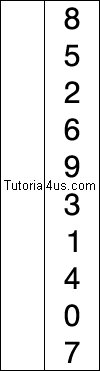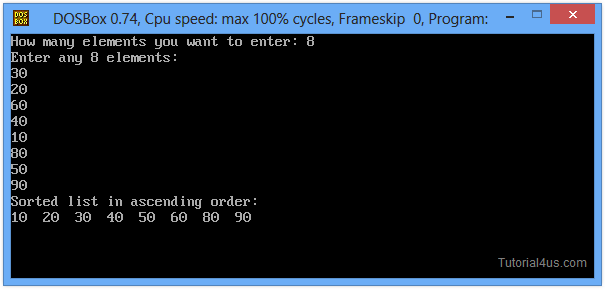# Selection Sort in C++

## Selection Sort Program in C++

Selection sort is based of maximum and minimum value. First check minimum value in array list and place it at first position (position 0) of array, next find second smallest element in array list and place this value at second position (position 1) and so on. Same process is repeated until sort all element of an array.

• Find the minimum element in the list.
• Swap it with the element in the first position of the list.
• Repeat the steps above for all remaining elements of the list starting from the second position.### Advantage of this sorting technique

It is very simple and easy method to sort elements.

### Dis-Advantage of this sorting technique

It is time consuming and slow process to sort elements.

## Selection Sort Program in C

```
#include<iostream.h>
#include<conio.h>

void main()
{
int array, n, c, d, position, swap;
clrscr();
cout<<"How many elements you want to enter: ";
cin>>n;
cout<<"Enter any"<<n<<"Elements: \n";

for (c=0; c<n; c++)
{
cin>>array[c];
}
for (c=0; c<(n-1); c++)
{
position=c;

for (d=c+1; d<n; d++)
{
if (array[position]>array[d])
{
position=d;
}
}
if (position!=c)
{
swap=array[c];
array[c]=array[position];
array[position]=swap;
}
}

cout<<"Sorted list in ascending order:\n";

for (c=0; c<n; c++)
{
cout<<array[c];
}
getch();
}
```

## Output

```
How many elements you want to enter: 8
Enter any 8  elements
5
3
6
2
7
8
1
4
Sorted list in ascending order : 1 2 3 4 5 6 7 8
```